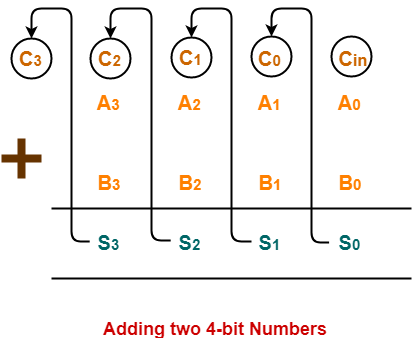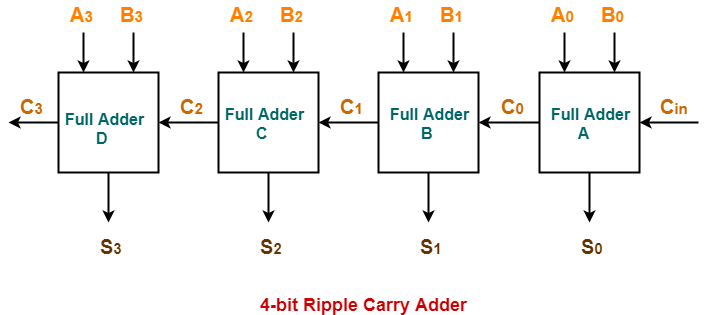• Ripple Carry Adder is a combinational logic circuit.
• It is used for the purpose of adding two n-bit binary numbers.
• It requires n full adders in its circuit for adding two n-bit binary numbers.
• It is also known as n-bit parallel adder.

 4-bit ripple carry adder is used for the purpose of adding two 4-bit binary numbers.

In Mathematics, any two 4-bit binary numbers A3A2A1A0 and B3B2B1B0 are added as shown below-Using ripple carry adder, this addition is carried out as shown by the following logic diagram-As shown-

• Ripple Carry Adder works in different stages.
• Each full adder takes the carry-in as input and produces carry-out and sum bit as output.
• The carry-out produced by a full adder serves as carry-in for its adjacent most significant full adder.
• When carry-in becomes available to the full adder, it activates the full adder.
• After full adder becomes activated, it comes into operation.

## Working Of 4-bit Ripple Carry Adder-

Let-

• The two 4-bit numbers are 0101 (A3A2A1A0) and 1010 (B3B2B1B0).
• These numbers are to be added using a 4-bit ripple carry adder.

4-bit Ripple Carry Adder carries out the addition as explained in the following stages-

## Stage-01:

• When Cin is fed as input to the full Adder A, it activates the full adder A.
• Then at full adder A, A0 = 1, B0 = 0, Cin = 0.

Full adder A computes the sum bit and carry bit as-

### Calculation of S0–

S0 = A0 ⊕  B0 ⊕ Cin

S0 = 1 ⊕ 0 ⊕ 0

S0 = 1

### Calculation of C0–

C0 = A0B0 ⊕  B0Cin ⊕ CinA0

C0 = 1.0 ⊕ 0.0 ⊕ 0.1

C0 = 0 ⊕ 0 ⊕ 0

C0 = 0

## Stage-02:

• When C0 is fed as input to the full adder B, it activates the full adder B.
• Then at full adder B, A1 = 0, B1 = 1, C0 = 0.

Full adder B computes the sum bit and carry bit as-

### Calculation of S1–

S1 = A1 ⊕  B1 ⊕ C0

S1 = 0 ⊕ 1 ⊕ 0

S1 = 1

### Calculation of C1–

C1 = A1B1 ⊕  B1C0 ⊕ C0A1

C1 = 0.1 ⊕ 1.0 ⊕ 0.0

C1 = 0 ⊕ 0 ⊕ 0

C1 = 0

## Stage-03:

• When C1 is fed as input to the full adder C, it activates the full adder C.
• Then at full adder C, A2 = 1, B2 = 0, C1 = 0.

Full adder C computes the sum bit and carry bit as-

### Calculation of S2–

S2 = A2 ⊕  B2 ⊕ C1

S2 = 1 ⊕ 0 ⊕ 0

S2 = 1

### Calculation of C2–

C2 = A2B2 ⊕  B2C1 ⊕ C1A2

C2 = 1.0 ⊕ 0.0 ⊕ 0.1

C2 = 0 ⊕ 0 ⊕ 0

C2 = 0

## Stage-04:

• When C2 is fed as input to the full adder D, it activates the full adder D.
• Then at full adder D, A3 = 0, B3 = 1, C2 = 0.

Full adder D computes the sum bit and carry bit as-

### Calculation of S3–

S3 = A3 ⊕  B3 ⊕ C2

S3 = 0 ⊕ 1 ⊕ 0

S3 = 1

### Calculation of C3–

C3 = A3B3 ⊕  B3C2 ⊕ C2A3

C3 = 0.1 ⊕ 1.0 ⊕ 0.0

C3 = 0 ⊕ 0 ⊕ 0

C3 = 0

Thus finally,

• Output Sum = S3S2S1S0 = 1111
• Output Carry = C= 0

### Why Ripple Carry Adder is Called So?

• The carry out produced by each full adder serves as carry-in for its adjacent most significant full adder.
• Each carry bit ripples or waves into the next stage.
• That’s why, it is called as “Ripple Carry Adder”.

• Ripple Carry Adder does not allow to use all the full adders simultaneously.
• Each full adder has to necessarily wait until the carry bit becomes available from its adjacent full adder.
• This increases the propagation time.
• Due to this reason, ripple carry adder becomes extremely slow.
• This is considered to be the biggest disadvantage of using ripple carry adder.

Watch this Video Lecture

Next Article- Delay in Ripple Carry Adder

Get more notes and other study material of Digital Design.

Watch video lectures by visiting our YouTube channel LearnVidFun.

SummaryArticle Name